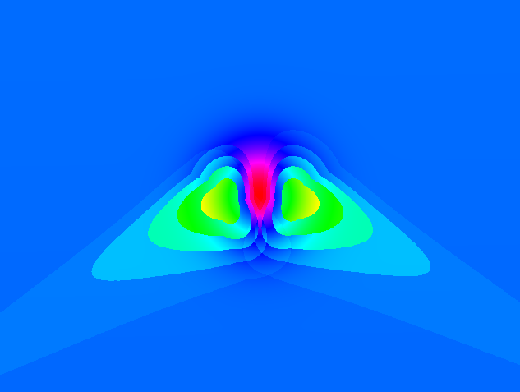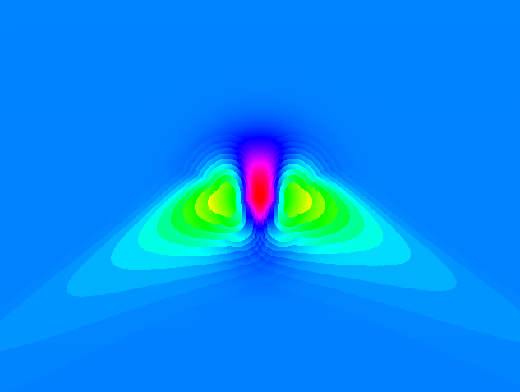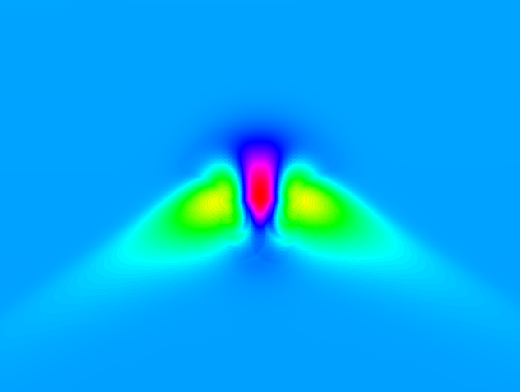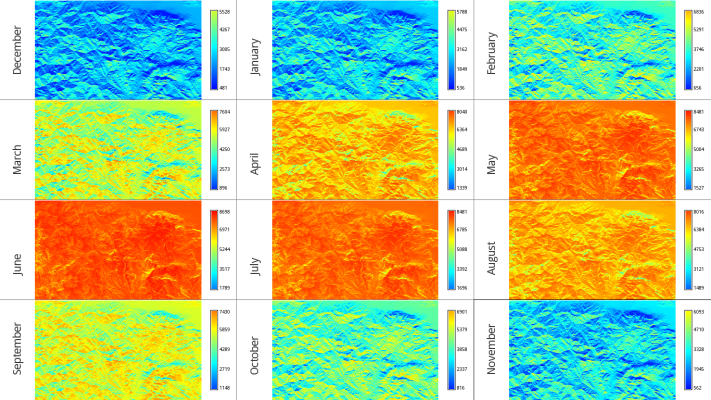# Difference between revisions of "R.sun"

## Tips

The speed of r.sun is much higher if r.horizon is used first and the resulting maps are given as input to r.sun. Background: the horizon needs to be computed only one time before, not in every step within r.sun. See the example at the end of the r.sun help page.

### Testing

Create an artificial surface containing a Gaussian mound:

```r.surf.volcano out=gauss method=gaussian kurtosis=1
```

Overlay some 200m contours to show underlying topography:

```r.contour in=gauss out=gauss_200m_contours step=200
d.vect gauss_200m_contours color=white
```

Set map's color table to highlight detail:

```r.colors rad_test.day355.beam col=bcyr -e
```

### Time step

The following three images demonstrate the effects of using different time step parameters.

```r.sun -s elevin="gauss" glob_rad="rad.global.30minT" day=180 step=0.5
```Default 30 minute time step over a Gaussian mound.

```r.sun -s elevin="gauss" glob_rad="rad.global.15minT" day=180 step=0.25
```15 minute time step over a Gaussian mound.

```r.sun -s elevin="gauss" glob_rad="rad.global.03minT" day=180 step=0.05
```3 minute time step over a Gaussian mound.

The 3 minute time step takes roughly ten times as long to run as the 30 minute timestep.

## Seed maps

Using seed maps can greatly (?, unclear) speed up processing. This is especially important if you will batch process e.g. for every day of the year.

• aspin= and slopein= options: create slope and aspect maps with the r.slope.aspect module.
Caution: currently buggy. See trac #498.
Estimated speedup:  ?%.

• horizon= and horizonstep= options: Pre-calculate horizon shadows by creating a series of horizon rasters with the r.horizon module.
Results not as smooth as without using this option?? See trac #498.
Estimated speedup:  ?%.

• latin= and longin= options: Pre-calculate latitudes and longitudes for each raster cell.

The following script will create a raster containing latitude as the raster value:

``` # create latitude map (WGS84)
g.region rast=elevation.dem
r.mapcalc one=1
r.out.xyz one | \
cut -f1,2 -d'|' | \
m.proj -oed --quiet | \
sed -e 's/[ \t]/|/g' | \
cut -f1-4 -d'|' | \
r.in.xyz in=- z=4 out=elevation.lat
g.remove one
```

Creating the longitude map is exactly the same but use column 3 of the m.proj output instead of column 4:

``` # create longitude map (WGS84)
g.region rast=elevation.dem
r.mapcalc one=1
r.out.xyz one | \
cut -f1,2 -d'|' | \
m.proj -oed --quiet | \
sed -e 's/[ \t]/|/g' | \
cut -f1-4 -d'|' | \
r.in.xyz in=- z=3 out=elevation.lon
g.remove one
```
Estimated speedup: 1.3% (in one test).
See trac #498.

The above assumes that your projection's ellipsoid is WGS84. If it isn't, use other proj_out= terms with m.proj as required instead of the -o flag.

## Automation

It can be tedious to set up and run a long series of r.sun simulations for every day of the year. To automate this we can write a small shell script loop:

``` for DAY in `seq 1 365` ; do
DAY_STR=`echo \$DAY | awk '{printf("%.03d", \$1)}'`
echo "Processing day \$DAY_STR at `date` ..."

r.sun -s elevin=elevation.dem day=\$DAY lin=\$LINKE step=0.05 \
done
```
• g.linke_by_day.py is a small program which will return the Linke coefficient for any day of the year, interpolated from monthly values. You need to edit the script to fill in values appropriate for your study area.
• If you have a multi-core CPU and you'd like to speed things up, here is a small Bourne shell script implementing a poor-man's multi-processing trick:
``` ### r.sun mode 2 loop ###
BEGIN=1
END=365
STEP=1
NUM_CORES=4

for DAY in `seq \$BEGIN \$STEP \$END` ; do
DAY_STR=`echo \$DAY | awk '{printf("%.03d", \$1)}'`
echo "Processing day \$DAY_STR at `date` ..."

CMD="r.sun -s elevin=elevation.dem day=\$DAY lin=\$LINKE step=0.05 \

# poor man's multi-threading for a multi-core CPU
MODULUS=`echo "\$DAY \$STEP \$NUM_CORES" | awk '{print \$1 % (\$2 * \$3)}'`
if [ "\$MODULUS" = "\$STEP" ] ; then
# stall to let the background jobs finish
\$CMD
sleep 2
#while [ `pgrep -c r.sun` -ne 0 ] ; do
#   sleep 5
#done
else
\$CMD &
fi
done
```
• For a r.sun Mode 1 loop, see the OpenMP page.

## Overview of monthly maps

Given there are mean monthly global irradiation maps calculated, an overview map of all 12 maps can be drawn along with their legends (see also Common legends for many raster maps). The script assumes a common naming convention for all 12 monthly average maps (i.e. global_rad_avg.jan, global_rad_avg.feb, global_rad_avg.mar, etc.).

``` #!/bash/sh
# script to draw monthly (mean) global solar irradiation maps in a 3x4 matrix

# set wide aspect ratio (16:9, e.g. 1366 width x 768 height)
d.monsize setm=x0 setw=1366 seth=768

# split in 12 frames
d.split.frame frames=12

# preferred font?
d.font DroidSans

for FRAME in "uno dec December" "dos jan January" "tres feb February" \
"cuatro mar March" "cinco apr April" "seis may May" \
"siete jun June" "ocho jul July" "nueve aug August" \
"diez sep September" "once oct October" "doce nov November"

do

# parse "\${FRAMES_STR}" and set positional parameters
set -- \$FRAME ; echo \$1 \$2 \$3

# select FRAME
d.frame -s "\${1}"

# draw the map

# draw label on the left and vertically
d.text text="\${3}" size=10 color=50:50:50 at=9,25 rotation=90

# draw legend

done
```Mean monthly global irradiation maps (n=38.918, s=38.531, w=21.793, e=22.436)

Same image also here (a bit of higher resolution)

## Development

### OpenCL

As part of the Google Summer of Code 2010 an OpenCL version has been written that allows r.sun to run on GPUs. This provides a massive speedup in processing time. The merging of this code into GRASS 7's r.sun is forthcoming (HB).

• To get your hands on the code now see http://github.com/mailseth/OpenCL-integration-for-GRASS---GDAL (functional prototype now available)
• To use OpenCL GPU functionality you'll want a graphics card like the ATI HD5770 or nVidia GTX260 or GTX460, or newer.
• OpenCL also allows for running on multi-core (or multi-processor) CPUs, for systems without GPUs. (Q: is it possible to do BOTH multicore CPU + your GPU?)
• Seth wrote: The OpenCL version of r.sun runs over 20x faster than the original version on my machine (2.26 GHz Mac Pro vs. GeForce GTX 285). However, it is hampered by the low memory on your GPU, so you may need to partition your raster.

## ToDo

• Merge in OpenCL code
• Add support for OpenMP parallelization (if OpenCL doesn't make that redundant)